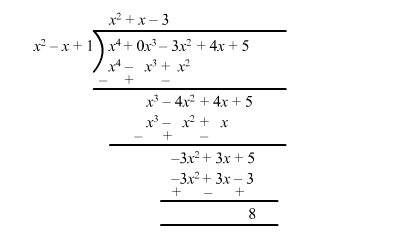# Find the quotient and remainder when:

Question:

Find the quotient and remainder when:

$f(x)=x^{4}-3 x^{2}+4 x+5$ is divided by $g(x)=x^{2}+1-x$

Solution:Quotient $q(x)=x^{2}+x-3$

Remainder $r(x)=8$